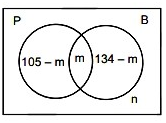### CAT 2019 – Slot 1 – Quantitative Ability – A trader sells 10 litres of a mixture of paints A and B

Q. 1:A trader sells 10 litres of a mixture of paints A and B, where the amount of B in the mixture does not exceed that of A. The cost of paint A per litre is Rs. 8 more than that of paint B. If the trader sells the entire mixture for Rs. 264 and makes a profit of 10%, then the highest possible cost of paint B, in Rs. per litre, is
1. 26
2. 16
3. 20
4. 22

Solution: let cost of paint A = x / liter

So cost of paint B = (x-8)/liter

Cost of 10 liter of the mixture = 264/1.1 = 240

Cost per liter of mixture = 240/10 = 24

For highest possible cost of paint B, both A and B should be in equal ratio , so

Cost of mixture = (x+ x -8)/2

24 = (2x -8)/2

x = 28

thus cost of paint B  = 28 -8 = 20 per liter

##### Q. 2: In a circle, two parallel chords on the same side of diameter have lengths 4 cm and 6 cm. If the distance between these chords is 1 cm, then the radius of the circle, in cm, is?1. √112. √133. √124. √14

√13

Q. 3: If among 200 students, 105 like pizza and 134 like burger, then the number of students who like only burger can possibly be
1. 93
2. 26
3. 23
4. 96

Let the number of students who like both pizza and burger be ‘m’ . The number of students who like neither of them be nFrom venn diagram 105 – m + m + 134 – m + n = 200 m – n = 39

∴ The possible values of (m, n) are (39, 0) (40, 1)…….(105, 66)

∴ The number of students who like only burger is lies in the range [134 – 105, 134 – 39] = [29, 95]

∴ From options, 93 is a possible answer

Q. 4: In an apartment complex, the number of people aged 51 years and above is 30 and there are at most 39 people whose ages are below 51 years. The average age of all the people in the apartment complex is 38 years. What is the largest possible average age, in years, of the people whose ages are below 51 years?
1. 27
2. 28
3. 26
4. 25

Let the average age of people aged 51 years and above be x years. Let the average age of people aged below 51 years be y years.

Let the number of people aged below 51 years be N.

Given, the average age of all the people in the apartment complex is 38 years.

Therefore,
(x*30+y*N) / 30+N = 38 ……(1)

We want to maximize y, which occurs when x is minimum i.e. for x=51. Substituting the value of x in (1) we get
390=N×(38-y)

Again, when y is maximum, N is also maximum i.e. 39 Therefore maximum value of y = 28.

Q. 5: Given an equilateral triangle T1 with side 24 cm, a second triangle T2 is formed by joining the midpoints of the sides of T1. Then a third triangle T3 is formed by joining the midpoints of the sides of T2. If this process of forming triangles is continued, the sum of the areas, in sq cm, of infinitely many such triangles T1, T2, T3,… will be?
1. 164√3
2. 188√3
3. 248√3
4. 192√3

As P,Q and R are mid points of AC,AB and BC so both triangle PQR and CBA will be similar and PQ = BC/2

So if the area of triangle ABC = A then area of triangle PQR = A/4

Thus

Sum of Areas = A+A/4+A/16+A/64+ ——–=A/(1-1/4)=4/3 A=4/3×√3/4×24^2=192√3

Inspiring Education… Assuring Success!!

Ghatkopar | Borivali | Andheri | Online